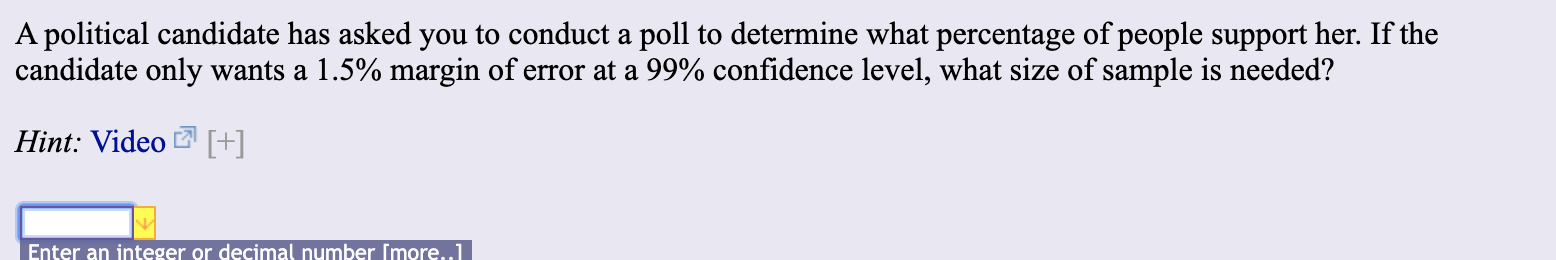# A political candidate has asked you to conduct a poll to determine what percentage of people support her. If thecandidate only wants a 1.5% margin of error at a 99% confidence level, what size of sample is needed?Hint: Video [+]Enter an integer or decimal number Imore..1

Question
25 viewshelp_outlineImage TranscriptioncloseA political candidate has asked you to conduct a poll to determine what percentage of people support her. If the candidate only wants a 1.5% margin of error at a 99% confidence level, what size of sample is needed? Hint: Video [+] Enter an integer or decimal number Imore..1 fullscreen
check_circle

Step 1

Sample size:

The minimum sample size for a desired confidence level, α with a given margin of error, E is given by:

Step 2

Computing the sample size:

Thus, it is given that the confidence level is 99%. Therefore, the significance level, α = 0.01.

From the EXCEL, using the formula, = NORM.INV(0.995,0,1), the critical value, zα/2 is 2.576.

That is, the true ...

### Want to see the full answer?

See Solution

#### Want to see this answer and more?

Solutions are written by subject experts who are available 24/7. Questions are typically answered within 1 hour.*

See Solution
*Response times may vary by subject and question.
Tagged in

### Hypothesis Testing